Subsections

## alignment.salign() — align two or more sequences/structures of proteins

salign(residue_type2='REGULAR', no_ter=False, overhang=0, off_diagonal=100, matrix_offset=0.0, gap_penalties_1d=(-900.0, -50.0), gap_penalties_2d=(3.5, 3.5, 3.5, 0.2, 4.0, 6.5, 2.0, 0.0, 0.0), gap_penalties_3d=(0.0, 1.75), feature_weights=(1.0, 0.0, 0.0, 0.0, 0.0, 0.0), rms_cutoff=3.5, fit=True, surftyp=1, fit_on_first=False, gap_function=False, align_block=0, max_gap_length=999999, align_what='BLOCK', input_weights_file=None, output_weights_file=None, weigh_sequences=False, smooth_prof_weight=10, fix_offsets=(0.0, -1.0, -2.0, -3.0, -4.0), substitution=False, comparison_type='MAT', matrix_comparison='CC', alignment_type='PROGRESSIVE', edit_file_ext=('.pdb', '_fit.pdb'), weights_type='SIMILAR', similarity_flag=False, bkgrnd_prblty_file='(LIB)/blosum62_bkgrnd.prob', ext_tree_file=None, dendrogram_file='', matrix_scaling_factor=0.0069, auto_overhang=False, overhang_factor=0.4, overhang_auto_limit=60, local_alignment=False, improve_alignment=True, fit_atoms='CA', output='', write_whole_pdb=True, current_directory=True, write_fit=False, fit_pdbnam=True, rr_file='(LIB)/as1.sim.mat', n_subopt=0, subopt_offset=0.0, align3d_trf=False, normalize_pp_scores=False, gap_gap_score=0.0, gap_residue_score=0.0, nsegm=2, matrix_offset_3d=-0.1, break_break_bonus=10000.0, io=None)
Output:
SalignData object

This command is a general dynamic programming based alignment procedure for aligning sequences, structures or a combination of the two. It is loosely based on the program COMPARER [Šali & Blundell, 1990]. SALIGN can be used to generate multiple protein structures/sequences alignments or to align two blocks of sequences/structures that are in memory.

See also Section 6.34 for utility scripts to simplify the high-level usage of SALIGN.

Please note that the method is still in development, and has not yet been fully benchmarked. As with any other alignment method, generated alignments should be assessed for quality.

Broadly classifying, three different types of protein alignment categories are tackled by this command:
1. Multiple structure alignments
2. Aligning a structure block to a sequence block
3. Multiple and pair-wise protein sequence alignment

The command incorporates the functionality of several old MODELLER commands (alignment.align(), alignment.align2d(), alignment.malign(), alignment.align3d(), and alignment.malign3d()). Some of the examples below illustrate the equivalent script files to replace the old alignment commands with alignment.salign().

In addition to these, this command has several new alignment features including profile-profile sequence alignments and a dendrogram based multiple sequence/structure alignment among others.

All pair-wise alignments make use of local or global dynamic programming. A switch from one to another can be effected by setting local_alignment to True or False. The dynamic programming can be carried out using affine gap penalties (as previously used in alignment.align(), by setting gap_function to False) or an environment dependent gap penalty function (as used in alignment.align2d(), by setting gap_function to True). (Please note that the default gap_penalties_1d parameters are optimal for the affine gap penalty; see the align2d examples for reasonable parameters if you wish to use the environment dependent gap penalty.) All arguments that associated to the alignment.align() and alignment.align2d() commands apply.

If at least one of the blocks in a pairwise alignment consists of structures, dynamic programming can be performed using structure dependent gap penalties.

On successful completion, an SalignData object is returned, from which some of the calculated data can be queried. For example, if you save this in a variable 'r', the following data are available:
• r.aln_score; the alignment score
• r.qscorepct; the quality score (percentage) if output contains 'QUALITY'

### Features of proteins used for alignment

Central to the dynamic programming algorithm is the weight matrix. In SALIGN, this matrix is constructed by weighting the contribution from six features of protein structure and sequence:

Feature 1
is the residue type.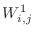is obtained from the residue type - residue type dissimilarity matrix, specified in the file rr_file.dissimilarity score for positions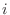and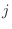in the two compared sub-alignments is the average dissimilarity score for a comparison of all residues in one sub-alignment with all residues in the other sub-alignment (note that gaps are ignored here). Should only feature weight 1 be non-zero, the user has an option of considering residue-residue similarity scores instead of distance scores by setting similarity_flag to True. (The other features are distance features, and so if their weights are non-zero, similarity_flag must be turned off, which is the default.)

Feature 2
is the inter-molecular distance for a pair of residues (unless align3d_trf is True: see alignment.align3d()). Only one atom per residue is of course selected, as specified by fit_atoms (e.g., Cα , although we should also allow for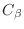in the future, which requires an intervention for Gly). This ‘position’ feature is complicated because it depends on the relative orientation of the structures corresponding to the two compared alignments.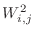is the Euclidean distance between the compared positionsandin the two compared sub-alignments that are already optimally aligned and superposed based on their coordinates alone. This optimal alignment is obtained by an iterative procedure as follows (the same as in alignment.align3d()). The average structures for both sub-alignments are calculated for all sub-alignment positions with at least one defined selected atom. This calculation is straightforward because the structures within the two sub-alignments are already superposed with each other (see below). Then, the distance matrix for dynamic programming with affine gap penalties is calculated as the matrix of Euclidean distances between the two averages. The dynamic programming results into a new alignment, dependent also on the gap initiation and extension penalties gap_penalties_3d (a reasonable setting is (0, 3)). gap_penalties_3d is a gap creation penalty (usually 0), and gap_penalties_3d is a gap extension penalty, say 3. When the gap initiation penalty is 0, pairs of positions are identified as equivalent when they have their selected atoms at most 2 times gap_penalties_3d angstroms apart in the current superposition, as described for the alignment.align3d() command. The new alignment is then used to generate the new superposition of the two averages, and the iteration of the distance matrix calculation, alignment and superposition is repeated until there are no changes in the number of equivalent positions and in the rotation matrix relating the two averages.

The values of both improve_alignment and fit are used in the calculation of the position feature. That is, the initial alignment and the orientation of the coordinates can be selected not to change at all during the calculation of the inter-molecular distance matrix.

When the calculation of the inter-molecular distance matrix is finished, all the structures in the second sub-alignment are rotated and translated following the optimal rotation and translation of the second average on the first average. These superpositions prepare the individual structures for the next of the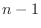stages of the progressive multiple alignment, and also orient all the structures for writing out to atom files with a '_fit.pdb' extension if write_fit = True. If fit_pdbnam = False, the PDB filenames in the output alignment file will not have the '_fit.pdb' extensions. Thus, feature 2 needs to be selected by feature_weight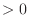if you wish to write out the structures superposed according to the tree-following procedure; also, fit_on_first must be False, otherwise the structures are written out superposed on the first structure according to the final alignment (see also below).

The alignment produced within the routine that calculates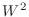does not generally correspond to the alignment calculated based on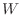. Therefore, the multiply superposed structures are not necessarily superposed based on the final multiple alignment produced by alignment.salign(). If you wish such a superposition, you can use alignment.malign3d() with fit = False and write_fit = True (the meaning of fit is different between alignment.salign() and alignment.malign3d()).

Unless the position feature is selected, the initial alignment does not matter. If the position feature is selected, a good starting alignment is a multiple sequence alignment, obtained either by alignment.malign() or by alignment.salign() used without the position feature (the initial alignment can also be prepared using the position feature). If the position feature is used, each pair of structures needs to have at least 3 aligned residues at all points during the alignment.

There are several possibilities as to the final orientation of the input coordinates. If fit_on_first is True, all the coordinate sets are superposed on the first structure, using the final multi-feature multiple alignment. If fit_on_first is False, and position feature was used, and fit was True, the coordinates will be superposed in the progressive manner guided by the tree, by the routine that calculates the inter-molecular distance matrices; this superposition is based only on the positions of the selected atoms (feature 2), not on other features such as residue type, secondary, structure, etc. If improve_alignment is False, it does not make much sense to have fit = True (use fit_on_first = True).

For local alignments, the matrix offset variable is matrix_offset_3d.

Feature 3
is the fractional sidechain accessibility. The pair-wise residue-residue dissimilarity is calculated by classifying residues into the buried (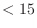%), semi-exposed, and exposed classes (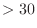%). The dissimilarity is 0 for equal classes or if the absolute difference in the accessibility is less than 5%, 1 for neighboring classes and 2 for the buried-exposed match. The position-position dissimilarity is the average residue-residue dissimilarity for comparing all residues from one group to all residues in the other group (gaps are ignored).

Feature 4
is the secondary structure type, distinguishing between helix, strand, and other. The pair-wise residue-residue dissimilarity is 0 for equal classes, 1 for ‘helix’ or ‘strand’ matched to ‘other’, and 2 for ‘helix’ matched to 'strand'. Position-position dissimilarity is calculated in the same way as for feature 3.

Feature 5
is the local conformation. A pair-wise residue-residue score is DRMSD between the selected atoms (fit_atoms) from the segments of (2*nsegm + 1) residues centered on the two matched residues. Position-position dissimilarity is calculated in the same way as for feature 3.

Feature 6
is a user specified feature for which a external matrix (in MODELLER matrix format; see the substitution matrices in the modlib directory for examples) has to be specified using input_weights_file. The user can input either a similarity matrix (weights_type = SIMILAR) or a distance matrix (weights_type = DISTANCE).

### Alignment of protein sequences

• Multiple protein sequence alignment
Aligning multiple sequences is similar to aligning multiple structures, the difference being that for sequence alignments only the first feature weight can be non-zero (the other features require coordinates). This is the default for feature_weights.

Example: examples/salign/salign_multiple_seq.py

# Illustrates the SALIGN multiple sequence alignment
from modeller import *

log.verbose()
env = environ()
env.io.atom_files_directory = ['.', '../atom_files']

aln = alignment(env, file='malign_in.ali')

aln.salign(overhang=30, gap_penalties_1d=(-450, -50),
alignment_type='tree', output='ALIGNMENT')

aln.write(file='malign.ali', alignment_format='PIR')


• Alignment of two sequence blocks
Two blocks of sequences can be aligned using the information contained within each of the multiple sequence blocks [Martı-Renom et al., 2004]. Pairs of sequence blocks are aligned using SALIGN the same way in which alignment.align() aligned sequence blocks; to align two blocks, simply proceed as normal, but set align_block to the number of sequences in the first block (the rest of the sequences are placed in the second block) and set alignment_what to BLOCK. Also, since this kind of alignment is effected only between two blocks, alignment_type is set to PAIRWISE.

• Alignment of protein sequences by their profiles
As for pairwise alignment of sequence blocks, above, two blocks can be aligned by their profiles. align_block demarcates the end of the first block and align_what is set to PROFILE indicating that the blocks will be aligned using their profiles, and alignment_type is set to PAIRWISE. The weight matrix for dynamic programming is created by comparing the sequence information in the two blocks. Two kinds of comparisons can be performed:
1. A correlation coefficient of the variation of the the 20 amino acids at each position (comparison_type is set to PSSM).
2. Comparing the residue substitution matrices implied at each position of the two blocks (comparison_type is set to MAT).
Matrix comparisons are of three types: taking the maximum, average or correlation coefficient of residue-residue substitution at the aligned positions (matrix_comparison set to MAX, AVE or CC).

Profile comparisons are done in similarity space rather than distance space, so similarity_flag should be set to True. They will also currently only work with feature 1 (since feature 1 is the only feature which works both in similarity and in distance space) - the weights for all other features must be set to zero.

Example: examples/salign/salign_profile_profile.py

# profile-profile alignment using salign
from modeller import *

log.level(1, 0, 1, 1, 1)
env = environ()

aln = alignment(env, file='mega_prune.faa', alignment_format='FASTA')

aln.salign(rr_file='{LIB}/blosum62.sim.mat', gap_penalties_1d=(-500, 0), output='', align_block=15, # no. of seqs. in first MSA align_what='PROFILE', alignment_type='PAIRWISE', comparison_type='PSSM', # or 'MAT' (Caution: Method NOT benchmarked # for 'MAT') similarity_flag=True, # The score matrix is not rescaled substitution=True, # The BLOSUM62 substitution values are # multiplied to the corr. coef. #output_weights_file='test.mtx', # optional, to write weight matrix smooth_prof_weight=10.0) # For mixing data with priors #write out aligned profiles (MSA) aln.write(file='salign.ali', alignment_format='PIR') # Make a pairwise alignment of two sequences aln = alignment(env, file='salign.ali', alignment_format='PIR', align_codes=('12asA', '1b8aA')) aln.write(file='salign_pair.ali', alignment_format='PIR') aln.write(file='salign_pair.pap', alignment_format='PAP')  ### Alignment of protein structures with sequences As stated earlier, all alignment.align() and alignment.align2d() related commands apply to alignment.salign() too. The example below is a alignment.salign() equivalent of alignment.align2d() (and alignment.align()). For a description of the gap_penalties_2d see the section on alignment.align2d(). Example: examples/salign/salign_align2d.py # align2d/align using salign # parameters to be input by the user # 1. gap_penalties_1d # 2. gap_penalties_2d # 3. input alignment file from modeller import * log.verbose() env = environ() env.io.atom_files_directory = ['../atom_files'] aln = alignment(env, file='align2d_in.ali', align_codes='all') aln.salign(rr_file='(LIB)/as1.sim.mat',  # Substitution matrix used
output='',
max_gap_length=20,
gap_function=True,              # If False then align2d not done
feature_weights=(1., 0., 0., 0., 0., 0.),
gap_penalties_1d=(-100, 0),
gap_penalties_2d=(3.5, 3.5, 3.5, 0.2, 4.0, 6.5, 2.0, 0.0, 0.0),
# d.p. score matrix
#output_weights_file='salign.mtx'
similarity_flag=True)   # Ensuring that the dynamic programming
# matrix is not scaled to a difference matrix
aln.write(file='align2d.ali', alignment_format='PIR')
aln.write(file='align2d.pap', alignment_format='PAP')


Caution: The values of gap_penalties_2d have been optimized for similarity matrices. If using a distance matrix, you will need to derive new optimized values.

### Alignment of protein structures

Structure alignments can make use of all the 5 structure/sequence features as well as the 6th user provided feature matrix. Pairwise alignments of structures can make use of the constant gap penalties or the environment dependent gap penalties. Multiple structure alignments are constructed from pairwise structure alignments.

This section describes the use of SALIGN to produce a single alignment of multiple structures. If the best output alignment is desired, it is recommended to run SALIGN in an iterative fashion, to determine the best parameter values. A utility script is provided for this purpose - see iterative_structural_align().

• Pairwise protein structure alignment
For optimal pairwise alignments it is suggested to call SALIGN multiple times, typically 2-3 times. The first SALIGN call will give an initial alignment which is refined in the subsequent calls. Usually, feature 2 is made non-zero only during the 'refinement' stage as rigid body refinement is done at the position implied by the alignment in memory.

• Tree Multiple Structure Alignments
When alignment_type is set to tree, a dendrogram of the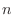proteins in memory is calculated using the selected features. The multiple alignment is then a progression ofpairwise alignments of the growing sub-alignments. A sub-alignment is an alignment of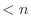proteins. The pairwise alignment of two sub-alignments is achieved using affine or environment dependent gap penalties, depending on whether gap_function is set to False or True (arguments to the alignment.align() and alignment.align2d() commands apply).

• Progressive Multiple Structure Alignments
If alignment_type is set to progressive, the multiple alignment follows only the last part of the ‘tree’ alignment where, inalignments, all the structures/sequences are successively aligned to the first one on the list.

The alignment of proteins within a sub-alignment does not change when the sub-alignment is aligned with another protein or sub-alignment. The pairwise alignment of sub-alignments is guided by the dendrogram. First, the most similar pair of proteins are aligned. Second, the next most similar pair of proteins are aligned, or the third protein is aligned with the sub-alignment of the first two, as indicated by the dendrogram. This greedy, progressive procedure requiressteps to align allproteins, and each step requires a pairwise alignment of two sub-alignments.

If in a multiple alignment, overhangs are to be penalized differently for the pairs of alignments that create the multiple, auto_overhang can be set to True. This will ensure that the value of overhang changes as overhang_factor times the numerical difference in the residues of the pair. Further, this is only effected if the difference is greater than overhang_auto_limit.

The dendrogram can be written out in a separate file by specifying the file name to dendrogram_file.

Example: examples/salign/salign_multiple_struc.py

# Illustrates the SALIGN multiple structure/sequence alignment
from modeller import *

log.verbose()
env = environ()
env.io.atom_files_directory = ['.', '../atom_files']

aln = alignment(env)
for (code, chain) in (('1is4', 'A'), ('1uld', 'D'), ('1ulf', 'B'),
('1ulg', 'B'), ('1is5', 'A')):
mdl = model(env, file=code, model_segment=('FIRST:'+chain, 'LAST:'+chain))
aln.append_model(mdl, atom_files=code, align_codes=code+chain)

for (weights, write_fit, whole) in (((1., 0., 0., 0., 1., 0.), False, True),
((1., 0.5, 1., 1., 1., 0.), False, True),
((1., 1., 1., 1., 1., 0.), True, False)):
aln.salign(rms_cutoff=3.5, normalize_pp_scores=False,
rr_file='(LIB)/as1.sim.mat', overhang=30, gap_penalties_1d=(-450, -50), gap_penalties_3d=(0, 3), gap_gap_score=0, gap_residue_score=0, dendrogram_file='1is3A.tree', alignment_type='tree', # If 'progresive', the tree is not # computed and all structues will be # aligned sequentially to the first #ext_tree_file='1is3A_exmat.mtx', # Tree building can be avoided # if the tree is input feature_weights=weights, # For a multiple sequence alignment only # the first feature needs to be non-zero improve_alignment=True, fit=True, write_fit=write_fit, write_whole_pdb=whole, output='ALIGNMENT QUALITY') aln.write(file='1is3A.pap', alignment_format='PAP') aln.write(file='1is3A.ali', alignment_format='PIR') # The number of equivalent positions at different RMS_CUTOFF values can be # computed by changing the RMS value and keeping all feature weights = 0 aln.salign(rms_cutoff=1.0, normalize_pp_scores=False, rr_file='(LIB)/as1.sim.mat', overhang=30,
gap_penalties_1d=(-450, -50), gap_penalties_3d=(0, 3),
gap_gap_score=0, gap_residue_score=0, dendrogram_file='1is3A.tree',
alignment_type='progressive', feature_weights=*6,
improve_alignment=False, fit=False, write_fit=True,
write_whole_pdb=False, output='QUALITY')


### Sub-optimal alignments

The weight matrix can be offset at random, many times over, to generate several ‘sub-optimal’ alignments. The number of sub-optimal alignments to be output can be specified with n_subopt. Though the matrix positions at which these offsets are applied cannot be controlled, the user can choose by how much the matrix will be offset (subopt_offset). The alignments are written into the file 'suboptimal_alignments.out' (or 'suboptimal_alignments2d.out' if gap_function is True) in a simple format. For each such alignment, an 'ALIGNMENT:' line is written, containing in order
• the number of the alignment (the first alignment is numbered 0, and is the true or 'optimal' alignment, for reference)
• the start, end and length of the alignment
• the number of aligned positions
• the score of the alignment
• three final fields for internal use
After this the two sequences are written as 'SEQ1' and 'SEQ2', as a simple mapping from alignment positions to sequences.

Note that subopt_offset should be positive for alignments using distance matrices (similarity_flag = False) and negative when using similarity matrices (similarity_flag = True).

Note that because the suboptimal alignments are generated sequentially, the alignment in memory at the end of the command will be the last (or worst) suboptimal alignment, not the optimal alignment.

The suboptimal alignment file can be converted into a set of real alignments using the alignment.get_suboptimals() method.

Example: examples/salign/salign_subopt.py

from modeller import *

log.verbose()
env = environ()
aln = alignment(env, file='fm07254_test.ali', alignment_format='PIR')
aln.salign(feature_weights=(1., 0, 0, 0, 0, 0), gap_penalties_1d=(-450, -50),
n_subopt = 5, subopt_offset = 15)

# Convert suboptimal alignment output file into actual alignments
f = open('suboptimal_alignments.out')
for (n, aln) in enumerate(aln.get_suboptimals(f)):
aln.write(file='fm07254_out%d.ali' % n)


### Alignments using external restraints

Fix positions: The user can choose to have certain alignment positions "fixed" by offsetting the appropriate matrix entries. This is done by adding a new pseudo sequence to the alignment with the align code ._fix_pos. The residues of this pseudo sequence are integer values from 0 through 4 (alternatively, a blank is equivalent to 0). Any alignment position at which this pseudo sequence contains a '0' is treated normally; if, however, a non-zero integer is used, the alignment matrix is offset, generally making the alignment in that position more favorable (and more so for higher integers). The actual offset values themselves can be specified by the user by setting the fix_offsets variable. Note that since SALIGN converts all DP scoring matrices to distance matrices (unless otherwise specified using similarity_flag), the values of fix_offsets used in anchoring alignment positions should be numerically smaller or negative in comparison to the values in the DP matrix.

Example: examples/salign/salign_fix_positions.py

# Demonstrating the use of alignment restraints, only available in
# align2d and salign:
from modeller import *

log.verbose()
env = environ()

# The special alignment entry '_fix_pos' has to be the last entry in the
# alignment array. Its sequence contains characters blank (or 0), 1, 2, 3,
# and 4 at the restrained alignment positions. The residue-residue score from
# the substitution matrix for these positions will be offset by the scalar
# value FIX_OFFSETS[0..4].
aln = alignment(env, file='fix_positions.ali', align_codes=('1leh', '3btoA',
'_fix_pos'))

# fix_offsets specifies the offset corresponding to character ' 1234' in the
# _fix_pos entry in the alignment
# (this offsets unlabeled positions for 0, the ones indicated by 1 by
#  1000, those indicated by 2 by 2000, etc.)
aln.salign(fix_offsets=(0, -10, -20, -30, -40),
gap_penalties_2d=(0, 0, 0, 0, 0, 0, 0, 0, 0), # Any values are
# possible here
local_alignment=False, # Local alignment works, too
gap_penalties_1d=(-600, -400)) # This is best with the default value
# of gap_penalties_2d

# Write it out, the _fix_pos is erased automatically in salign:
aln.write(file='fix_positions_salign.pap', alignment_format='PAP')


External weight matrix: An example of using feature 6.

Example: examples/salign/salign_external_matrix.py

# Reads an external matrix
from modeller import *

log.verbose()
env = environ()

aln = alignment(env, file='1dubA-1nzyA.ali', align_codes='all')

aln.salign(alignment_type='pairwise', output='',
rr_file='$(LIB)/blosum62.sim.mat', #rr_file='$(LIB)/as1.sim.mat',
#max_gap_length=20,
gap_function=False,
input_weights_file='external.mtx',   # External weight matrix
#weights_type='DISTANCE',            # type of ext. wgt. mtx
# ensure appropriate gap penalites for the ext. matrix
#feature_weights=(1., 0., 0., 0., 0., 0.), gap_penalties_1d=(30, 26),
#output_weights_file='score.mtx',
feature_weights=(1., 0., 0., 0., 0., 1.),
gap_penalties_1d=(-500, -300))
aln.write(file='output.ali', alignment_format='PIR')
aln.write(file='output.pap', alignment_format='PAP')


Multiple structure alignment according to a user specified dendrogram The user has the option of inputting anXmatrix from which a dendrogram can be inferred. The multiple tree alignment is then confined to follow this externally input dendrogram. To effect this, specify the name of the external matrix file with the ext_tree_file variable. The format of this file is the same as the external weight matrix, above; the first line of the file contains the dimensions of the matrix (in this case it should be a square matrix, with both dimensions equal to the number of sequences in the alignment), and subsequent lines contain sequence-sequence distances, one row of the matrix per line.

### Gap penalties and correcting for gaps

SALIGN makes use of three sets of gap penalties. gap_penalties_1d are for dynamic programming making use of constant gap penalties. gap_penalties_2d are when a variable function for gap penalty is used. gap_penalties_3d is used along with feature 2 only, when structures are aligned by a least squares fit of their atomic positions. All SALIGN features produce some measure of residue equivalence (similarity or distance scores). The scales of these scores differ depending on the feature used. For optimal usage, gap_penalties_1d should be set appropriately considering the features used. Note: If feature 1 is non zero and a similarity substitution matrix is employed, no matter what other features are also used in conjunction, gap_penalties_1d should always take on values appropriate to the substitution matrix used. For example, if feature 1 is non zero (other features may or may not be non-zero), and the residue substitution matrix used is the BLOSUM62 similarity matrix, gap_penalties_1d is set to (-450, -50) and when feature 1 is zero gap_penalties_1d is set to values appropriate for a distance matrix, e.g., (2, 3). A word of caution: gap penalties have not yet been optimized for aligning sequences by their profiles and for structure alignments.

The gap correction function is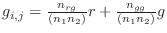, where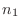and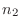are the number of proteins in the two sub-alignments,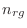is the number of gap-residue pairs, and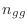is the number of gap-gap pairs when comparing protein positions from one sub-alignment with protein position from the other sub-alignment,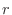is gap_residue_score and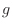is gap_gap_score. The smaller (even negative) is gap_gap_score, and the larger is gap_residue_score, the more will the gaps be aligned with gaps.

Whenever an alignment of two sequences is done and feature 1 is non-zero, SALIGN will attempt to keep any chain breaks aligned. This behavior can be tuned by setting the break_break_bonus parameter. See alignment.align() for more information.

### Useful SALIGN information and commands

The alignment.salign() command uses position-position dissimilarity scores (except when similarity_flag is switched on), as opposed to similarity scores. This convention applies to all the features, including the residue-residue similarities read from the rr_file; however, if a residue type - residue type similarity matrix is read in, it is automatically converted into the distance matrix by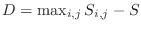. In addition, it is also scaled linearly such that the residue-residue dissimilarity scores range from 0 to 1 (to facilitate weighting this feature with other features).

For each pairwise alignment, the weight matrixhas dimensions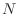and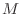that correspond to the lengths of the sub-alignments to be aligned based on the weight matrix. The dissimilarity score for aligning positionwith positionis calculated as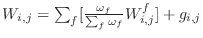, where the sum runs over all selected features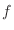, andis a function that may be used to correct the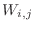score for the presence of gaps within the sub-alignments (see below). A featureis selected when its weight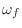(specified in feature_weights) is non-zero. The matrices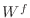are normalized to have the mean of 0 and standard deviation of 1 when normalize_pp_scores is True, but it is recommended not to use this option for now (i.e., use feature_weights to scale the contributions of the different features to the final). The weights of 1 will weigh the different features approximately evenly (the residue-residue dissimilarities of feature 1 are scaled to a range from 0 to 1, the position differences of feature 2 are in angstroms, the fractional solvent accessibility scores of feature 3 and the secondary structure scores of feature 4 range from 0 to 2, and the DRMS difference of feature 5 is expressed in angstroms).

If you enable verbose logging with log.verbose(), there will be more output in the 'log' file, such as the dendrogram. The dendrogram can also be written out in a separate file by specifying the file name to dendrogram_file.

Argument output can contain the following values:

• 'ALIGNMENT': the alignments in the first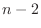stages of the pairwise alignment of sub-alignments are written out.

• 'QUALITY': the final alignment is used to obtain pairwise least-squares superpositions and the corresponding average and minimal numbers of pairs of aligned residues that are within rms_cutoff Å in all pairs of aligned structures. These numbers can be used as absolute quality measures for the final multiple alignment. This option requires the coordinate files for the aligned proteins.

If write_fit is True, the fitted atom files are written out in their fitted orientations. For this and other options below, also read the text above.

If output_weights_file is specified, the dynamic programming weight matrix is written out into the file. (If it is None, no file is written out.)

If current_directory is True, the output _pdb.fit files will be written to the current directory. Otherwise, the output will be in the directory with the original files6.9.

If write_whole_pdb is True, the whole PDB files are written out6.10; otherwise only the parts corresponding to the aligned sequences are output.

If fit is False, the initial superposition is not changed. This is useful when all the structures have to be compared with a given alignment as is, without changing their relative orientation.

If fit_on_first is True, the structures are fit to the first structure according to the final alignment before they are written out.

If improve_alignment is False, the initial alignment is not changed, though the structures may still be superimposed if fit = True. This is useful when all the structures have to be superimposed with the initial alignment.

Automatic builds 2019-06-19